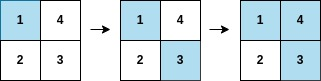2661. First Completely Painted Row or Column
Medium
263
5

You are given a 0-indexed integer array `arr`, and an `m x n` integer matrix `mat`. `arr` and `mat` both contain all the integers in the range `[1, m * n]`.

Go through each index `i` in `arr` starting from index `0` and paint the cell in `mat` containing the integer `arr[i]`.

Return the smallest index `i` at which either a row or a column will be completely painted in `mat`.

Example 1:```Input: arr = [1,3,4,2], mat = [[1,4],[2,3]]
Output: 2
Explanation: The moves are shown in order, and both the first row and second column of the matrix become fully painted at arr.
```

Example 2:```Input: arr = [2,8,7,4,1,3,5,6,9], mat = [[3,2,5],[1,4,6],[8,7,9]]
Output: 3
Explanation: The second column becomes fully painted at arr.
```

Constraints:

• `m == mat.length`
• `n = mat[i].length`
• `arr.length == m * n`
• `1 <= m, n <= 105`
• `1 <= m * n <= 105`
• `1 <= arr[i], mat[r][c] <= m * n`
• All the integers of `arr` are unique.
• All the integers of `mat` are unique.
Accepted
15.3K
Submissions
30.5K
Acceptance Rate
50.0%

Seen this question in a real interview before?
1/4
Yes
No

Discussion (0)

Related Topics